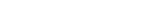MathExamle.com# Multiplication and Division of fractions

Create worksheets of mathematical examples with multiplication and division of integers and fractions, select type of a generator and viewing some samples of task

Creates examples with multiplication and division of fractions

3 Variants 3 Levels
 1 × 1 1 × 8 1 = 3 2 3
 4 2 ÷ 1 1 × 3 = 3 6 4
Variant: 2 Level: Easy

Creates expressions with fractions which need to reduce

1 Variant 3 Levels
 507 = 651
 10782 = 1422
Variant: 1 Level: Hard